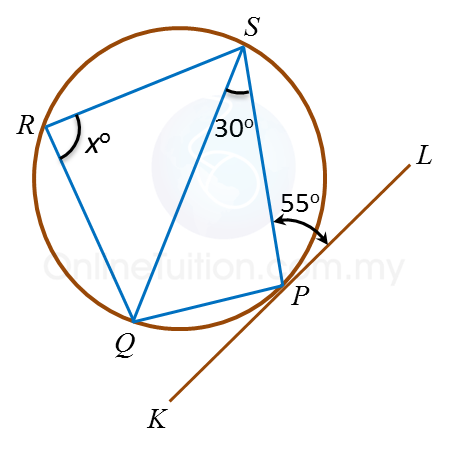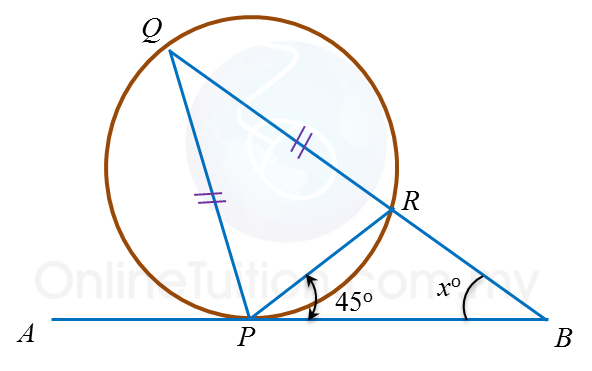# 6.7.2 Angles and Tangents of Circles, PT3 Focus Practice

 
Question 4:In figure above, PAQ is a tangent to the circle at point A. AEC and BED are straight lines. The value of y is
 

 
Solution:
 
ABD = ∠ACD = 40o
 
ACB = ∠PAB = 60o
 
y= 180– ∠ACB – ∠CBD – ∠ABD
 
y= 180– 60o – 25o– 40o = 55o
 
 
 
Question 5:In figure above, KPL is a tangent to the circle PQRS at point P. The value of x is
 
 

  Solution:
 
PQS = ∠SPL= 55o
 
SPQ = 180– 30o – 55o= 95o
 
 
SPQ + ∠SRQ = 180o
 
95o+ xo = 180o
 
x = 85o
 
 
 
Question 6:In figure above, APB is a tangent to the circle PQR at point P. QRB is a straight line. The value of x is
 
 
 
Solution:
 
PQR = ∠RPB = 45o
 
QPR = (180– 45o) ÷ 2 = 67.5o
 
PQR + ∠BPQ + xo = 180o
 
45+ (67.5o + 45o) + xo = 180o
 
 
x = 22.5o